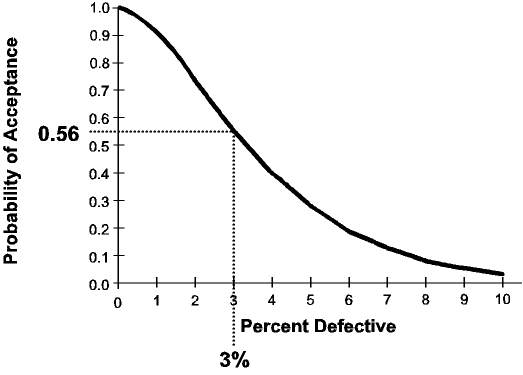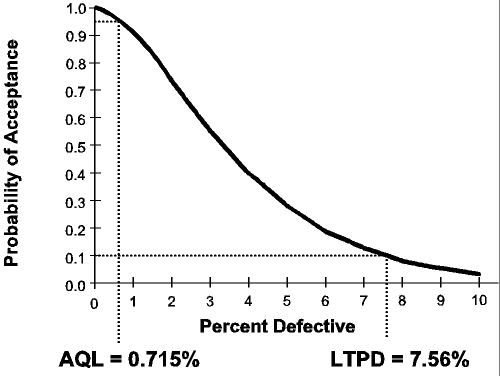# Confidence Statements Associated With Sampling Plans

Suppose the single sampling plan with sample size n=50 and accept number a=1 is being used.  If a lot is accepted, one can state with 90% confidence that the lot is less than 7.56% defective.  Likewise, if the lot is rejected, one can state with 95% confidence that the lot is above 0.715% defective.

The values 0.715 and 7.56 correspond to the AQL and LTPD0.10 of the sampling plan respectively.  These values are obtained from the operating characteristic (OC) curve of the sampling plan.  The figure below shows the OC curve of the above sampling plan.The bottom axis is the percent defective.  The left axis gives the corresponding probability of acceptance.  For example, a 3% defective lot has a 0.56 probability of acceptance. Each sampling plan has its own distinctive OC curve.

The AQL and LTPD0.10 represent two points on the OC curve.  The AQL is defined to be that percent defective with a 95% chance of acceptance. The following figure shows that for the single sampling plan n=50 and a=1, the AQL is 0.715% defective.  The AQL represents a level of defects routinely accepted by the sampling plan.Whenever a sampling plan rejects a lot, one can state with 95% confidence that the lot is above the AQL.  This is a result of the fact that lots at or below the AQL are not likely to be rejected.  The level of confidence, 95%, is a direct result of the fact that at the AQL there is a 95% chance of acceptance.

The LTPD0.10 is defined to be that percent defective with a 10% chance of acceptance.  The above figure shows that for the single sampling plan n=50 and a=1, the LTPD0.10 is 7.56% defective.  The LTPD0.10 represents a level of defects routinely rejected by the sampling plan.

Whenever a sampling plan accepts a lot, one can state that with 90% confidence that the lot is below the LTPD0.10.  This is a result of the fact that lots at or above the LTPD0.10 are not likely to be accepted.  The level of confidence, 90%, is equal to 100% – 10% where 10% is the chance of acceptance at the LTPD0.10.

The AQL and LTPD0.10 represent special cases of percentiles of the OC curve.  The AQL is the 95th percentile while the LTPD0.10 is the 10th percentile.  Other percentiles can be used as well. The LTPD0.05 is the 5th percentile. If a lot is accepted, one can state with 95% confidence that the lot is below the LTPD0.05.

The percentiles of sampling plans from MIL-STD-105E and ANSI/ASQC Z1.4 can be obtained from Table X of those standards.  Most other tables of sampling plans provide similar information.  The software package Sampling Plan Analyzer can also be used to determine the percentiles.

It is important to note that passing a sampling plan does not mean that the lot is “good.”  If we consider lots above the AQL as bad and lots below the AQL as good, then rejecting a lot proves that the lot is bad.  However, passing the lot only proves that the lot is below the LTPD, not the AQL.  Sampling plans will accept some lots above the AQL if such lots are produced.

Appeared in FDC Control, Food Drug & Cosmetic Division ASQ, No. 116, Dec. 1997, p. 2